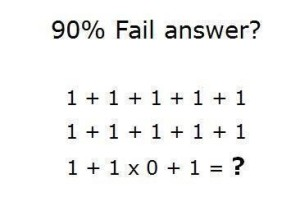# Category Archives: Geek

## Yep, Pretty Much

From my experience most devs think they are quite awesome except when they think they totally suck. This is the same person from minute to minute.

~ Mark Flory via Quora

Today on LinkedIn, I saw this:The following assumes:

• + is the arithmetic addition operator
• x is the arithmetic multiplication operator
• Standard order of operations: multiplication takes precedence

Given no line has operators joining them, an answer (in decimal) is:

• Line 1: 5
• Line 2: 5
• Line 3: 2

However, if one were to concatenate the lines (assuming whitespace is ignored), this gives:

1 + 1 + 1 + 1 + 11 + 1 + 1 + 1 + 11 + 1 x 0 + 1

Since there is no indication of which base is used, here are some answers:

• binary: 1110
• octal: 33
• decimal: 35